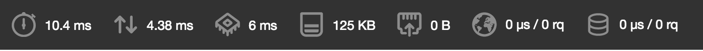# Quick Tip: Convenience Hacks for Passing Data to Views

In MVC based architectures, working with template engines is an inevitable part of the development routine. It usually goes like this: we prepare and pass the data to the view. In the view, we print them based on our layout design.Here is a basic example of how it works using Twig.

``````<?php

// Twig initialization

// Preparing data

\$user     = 'user data';
\$posts    = 'posts';

\$twig->render('author.page', [

'user'     => \$user,
'posts'    => \$posts,

]);

// ...
``````

There are times, however, when the number of variables might be much higher than this: ten or more. In that case, we’ll have a tall list of variables (as an associative array), being passed to the respective template. It gets messy and unreadable quickly. If only there was a way to just list what we need by name, and have PHP take care of the rest for us. Well… there is!

In this post, we’re going to talk about a trick for passing the defined variables to the view in a somewhat more convenient way: we can use the PHP native compact() function to handpick the data we need, by referring to the variables by name:

``````<?php

// Twig initialization

// Preparing data

\$user     = 'user data';
\$posts    = 'posts';

\$twig->render('author.page', compact('user', 'posts'));

// ...
``````

`compact()` accepts a list of names, looks for variables with those names (within the current scope) and returns them all as one associative array.

Sometimes it’s even more convenient to specify the variables we don’t need instead of those we do. Since there’s no built-in solution in PHP to compact all the variables (within the current scope) with a few of them excluded, we have to do this as a three-step process.

First we need to get all the variables within the controller’s scope with `get_defined_vars()`:

``````<?php
// ...
\$variables = get_defined_vars();
// ...
``````

Then, we filter out the unwanted variable names from wanted ones, by using PHP’s array_diff function.

`array_diff` compares an array against one or more arrays, and returns the entries in the first array, which are not present in any of the other arrays.

``````<?php
// ...
\$variables = get_defined_vars();
\$including = array_diff(array_keys(\$variables), ['user', 'posts', 'comments']);
// ...
``````

Finally, we can extract our desired variables (listed in `\$including`) out of `\$variables` by using `array_intersect_key()`.

`array_intersect_key()` accepts a number of arrays and returns the entries of the first array the keys of which exist in all the other arrays.

Please note since `array_intersect_key()` compares keys to get the intersection of the arrays, we need to switch the keys with their associated values in `\$including` by using array_flip():

``````<?php
// ...
\$variables = get_defined_vars();
\$including = array_diff(array_keys(\$variables), ['user', 'posts', 'comments']);

\$vars = array_intersect_key(\$variables, array_flip(\$including));
// ...
``````

Now, to make the procedure reusable, we can abstract the complexity into a helper function:

``````<?php

//Helpers.php

// ...

if (!function_exists('only_compact')) {

function only_compact(\$values, \$keys) {
\$keys = array_diff(array_keys(\$values), \$keys);
return array_intersect_key(\$values, array_flip(\$keys));

}
``````

And this is how it will make things easier:

``````<?php

// Twig initialization...

// Preparing data

\$user          = 'user data';
\$posts         = 'posts';
\$anotherOne    = 'some value';
\$yetAnotherOne = 0;
\$andAnotherOne = 0;
\$counter       = 0;
\$test_1        = 1;
\$test_2        = 2;
\$test_3        = 3;
\$test_4        = 4;
\$test_5        = 5;
\$test_6        = 6;

// Even more variables ...

\$twig->render('author.page', only_compact(get_defined_vars(), ['counter', 'twig']));
);

// ...
``````

As a result, we get an array of variables with all the unwanted variables excluded.

To use the helper file, we can add it under `files` (under `autoload`) in `composer.json`:

``````// ...

"files": [
"helpers.php"
]
}

// ...
``````

Since we’re using PHP’s built-in functions, the performance impact isn’t noticeable. Below is the result of a quick profile test done with Blackfire, before and after using the above techniques:

Without using the functions:With using the functions:As you can see, the results of both profile runs are almost the same.

Hopefully, this trick will save you some typing!

Have any other tips to share in regards to templating and view efficiency? Let us know!